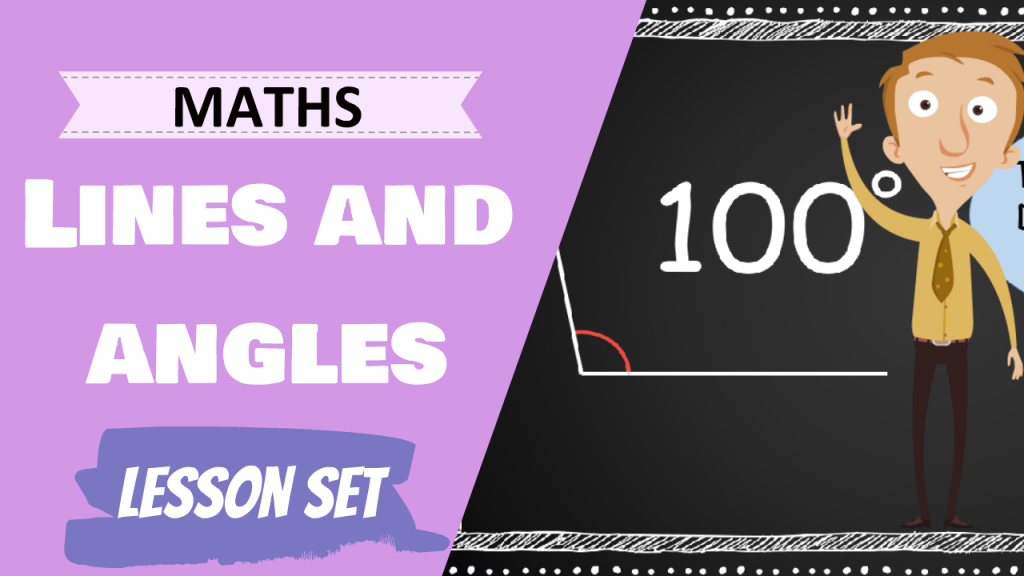# Lines and Angles# Lessons and Activities

### Lesson 1

Triangles and Angles
In this lesson, we'll take a look at how to estimate, measure and draw angles

### Lesson 2

Types of Lines and Angles
In this lesson, we'll take a look at how measure angles on a straight lines and a point

### Lesson 3

Lines of Symmetry
In this lesson, we'll take a look at how to recognize lines of symmtry in a shape or a pattern.

### Lesson 4

Using Diagonal Lines
In this lesson, we'll take a look at how to identify, measure and draw types of lines